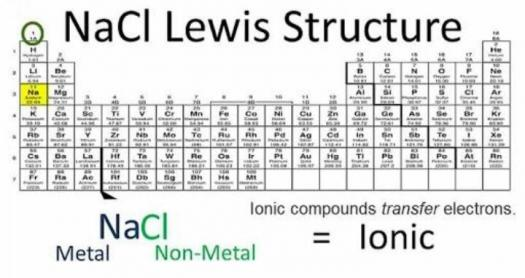# What Do You Know About Lewis Dot Diagrams?

10 Questions | Total Attempts: 180SettingsRegardless of whether you call them Lewis structures, electron dot structures, Lewis electron dot structures, Lewis dot structures, Lewis dot diagrams, or Lewis dot formulas, it wouldn't make any difference in light of the fact that the utilization is indistinguishable. They are illustrations utilized to demonstrate a specific bonding concept in the chemistry branch of science. To discover more, take this short test.

Related Topics
• 1.
What is another term for Lewis dot diagrams?
• A.

Electron photon structures

• B.

Electron bond structures

• C.

Electron atom structures

• D.

Electron dot structures

• 2.
When was the award introduced?
• A.

1916

• B.

1920

• C.

1926

• D.

1930

• 3.
Which of these represents a shared pair of electron in a Lewis dot structure?
• A.

Dot

• B.

Point

• C.

Line

• D.

Circle

• 4.
What does a Lewis structure represent between atoms of a molecule?
• A.

Heat

• B.

Transfer

• C.

Bonding

• D.

Energy

• 5.
As what must each electron first be placed?
• A.

Lone pairs

• B.

Valence electrons

• C.

Absolute pairs

• D.

Covalent bonds

• 6.
In form of which of these does a valence electron absorb or release energy?
• A.

Dot

• B.

Photon

• C.

Proton

• D.

Electron

• 7.
How many valence electrons have the boron group?
• A.

3

• B.

5

• C.

7

• D.

10

• 8.
What represents quantum number?
• A.

Q

• B.

N

• C.

X

• D.

Z

• 9.
How many valence electrons has phosphorus?
• A.

5

• B.

10

• C.

15

• D.

20

• 10.
Which is a noble gas?
• A.

Argon

• B.

Lithium

• C.

Oxygen

• D.

Hydrogen